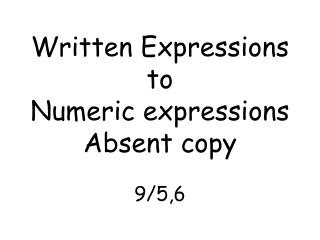DownloadDownload PresentationWritten Expressions to Numeric expressions Absent copy 9/5,6

# Written Expressions to Numeric expressions Absent copy 9/5,6

Télécharger la présentation## Written Expressions to Numeric expressions Absent copy 9/5,6

- - - - - - - - - - - - - - - - - - - - - - - - - - - E N D - - - - - - - - - - - - - - - - - - - - - - - - - - -
##### Presentation Transcript

1. Written Expressions toNumeric expressionsAbsent copy9/5,6

2. Word phrases that associate/w the four operations AdditionSubtraction Multiplication Division Multiply Divide Product Quotient Times • Add Subtract • Sum Difference • Plus Minus • Total Remainder • More than Less than • Increased by Decreased by Quantity = Mult. With parenthesis

3. Write a variable expression for the word phrase Answers • T + 9 2. Q - 16 3. X - 12 40 4. 5(7 + F) A number T increased by nine Sixteen less than a number Q A number X decreased by twelve, divided by forty Five times the quantity of 7 plus the number F.

4. Consecutive Whole numbers • The greatest of three consecutive whole numbers, the smallest of which is F • The smallest of four consecutive whole numbers, the biggest of which is K + 6 • Answers • 1 F, F + 1, F + 2 • 2 K + 6, K + 5, K + 4, K + 3